Motion couplers and momentum converters
Coyright 2004, 2010 by Brian Fraser
revised 2-5-2010n+

Electromagnetic science is full of  non-intuitive conceptsthings that seem contrary to common sense and what you were taught in high school physics. Consider, for example, how two charged particles interact when one is moving and the other is not (or when they are on perpendicular trajectories):

"We pointed out in Section 26-2 the failure of the law of action and reaction when two charged particles were moving on orthogonal trajectories. The forces on the two particles don't balance out, so the action and reaction are not equal; therefore the net momentum of the matter must be changing. It is not conserved. But the momentum in the field is also changing in such a situation. If you work out the amount of momentum given by the Poynting vector, it is not constant.. However, the change of the particle momenta is just made up by the field momentum, so the total momentum of particles plus field is conserved." (The Feynman Lectures on Physics, Vol. 2,  p. 27-11)

Newton's law of action/reaction does not apply in certain situations in electromagnetics, because the "force" is actually canceling a motion instead of producing one. This allows other motions to become manifest. To get the total picture, you have to look at what happens not only to the particles, but to the rest of the situation, something we currently call the "electromagnetic field". And because we live on a gravitationally bound reference system that is actually moving multidimensionally at the speed of light, things get even weirder when particles move at high speeds.  See The Feynman Lectures on Physics, Vol. 2, section 13-6 "The relativity of magnetic and electric fields" and chapter 26, "Lorentz Transformation of the Fields".

In the previous section you saw how motion of the wire in space produces mechanical energy. I further proposed that motion of space in the wire also produces energy, but of a different sort, namely electrical energy with an attendant magnetic field. Let us suppose now that we replace linear motion with rotational motion for these cases. Do we still get a picture that is self-consistent? Can we still extract energy from either system?  More particularly, can we extract momentum? Energy has only a magnitude, but momentum has both a magnitude and a direction.  Seeing what is going on with momentum would be even more illuminating than seeing what is happening with the energy.

To illustrate the issues, consider the behavior of the device shown in the illustration below. The marbles are revolving at high speed in a frictionless tube which is anchored to a turntable. The turntable is initially stationary, but is able to rotate freely. The system clearly has angular momentum, but if the tube is opaque, this is not obvious to an external observer. The observer can command the gate valve to close however. When it suddenly closes, the marbles will stop "flowing" in the tube and collide with the gate valve, which is securely mounted on the turntable. This rotational equivalent of "water hammer" will cause the turntable to start rotating.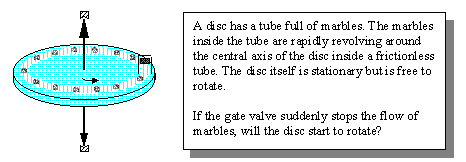Now suppose we have a coil of wire (a solenoid)  with current circulating through it instead of a tube with marbles. According to the "Motion Cancellers" discussion on the previous page, this system will likewise have angular momentum, but in this case, it is caused by space rotating in the wire, instead of the wire rotating in space. Suppose we command the battery to disconnect. The electric current must suddenly stop. The space revolving in the wire (electric current) comes to a halt. What then happens to the alleged angular momentum of the system? Does it just disappear? Or does it cause the turntable to rotate as in the previous (mechanical) example?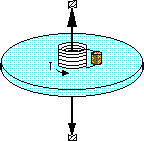I can tell you from personal experience what will happen. You'll see a big, fat spark when the battery disconnects, but the turntable will not rotate.

As a kid I used to play with inductors and batteries. I would connect a battery to a couple of wires from an old audio transformer, or a couple of wires from an old fluorescent light ballast. I noticed that some hookups would produce a little spark, some would produce a big, fat snappy spark, and others would produce nothing. When I got a couple of fingers across the terminals and disconnected the wire, I would sometimes get one heck of a shock. How could a little 6 volt battery and a little coil of wire produce such a high voltage?

Years later I would learn about V = L(dI/dt). This says that the voltage across an inductor is proportional to the time rate of change of the electric current. Disconnecting the wire caused the current (I) to change very suddenly. The time derivative of this is numerically large, and this causes the high voltage and fat spark. The energy which is stored in the (now) collapsing magnetic field suddenly returns to the wire.

Electrical engineers will tell you that  inductors act like an electrical flywheel and capacitors act like an electrical storage tank  Either can store considerable energy. When you disconnect a capacitor from a battery, you leave it with a "full tank of electric fluid"  (so to speak). The energy remains stored, and  does not have to go anywhere. But when you disconnect an inductor, the "flywheel" suddenly stops, and you get the electrical equivalent of water hammer. This is usually not desirable, and protective devices are inserted into circuits to dissipate the high voltage pulse. A diode, or a small lamp, for instance, will allow the current to circulate momentarily and come to a gradual stop even when the battery is disconnected suddenly.

So this little experiment demonstrates that an inductor stores energy, but does not specifically demonstrate storage of angular momentum. Mechanical momentum and electrical momentum still seem to be rather separate concepts. Yet according to the Motion Cancellers discussion (which you read previously) these should be equivalent. We should be able to show, without "cheating", that the electrical angular momentum can be turned into mechanical angular momentum by using fundamental electromagnetic principles directly, and without interposing some sort of energy conversion device.

This is NOT an intuitively easy problem to solve. However, the Poynting vector, and Feynman's comments about it, will serve to educate our intuition: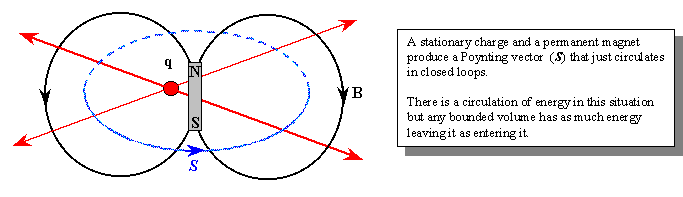"Suppose we take the example of a point charge sitting near the center of a bar magnet, as shown in [the figure]. Everything is at rest, so the energy is not changing with time. Also, E and B are quite static. But the Poynting vector says that there is a flow of energy, because there is an E X B that is not zero. If you look at the energy flow, you find that it just circulates around and around. There isn't any change in the energy anywhereeverything which  flows into one volume flows out again. It is like incompressible water flowing around. So there is a circulation of energy in this so-called static condition. . . .

You no doubt begin to get the impression that the Poynting theory at least partially violates your intuition as to where energy is located in an electromagnetic  field. . . . The circulation of energy around a magnet and a charge seem, in most circumstances, to be quite unimportant. It is not a vital detail, but it is clear that our ordinary intuitions are quite wrong." (The Feynman Lectures on Physics, Vol. 2, p. 27-8)

With that in mind, consider a little gizmo Feynman describes. It is very similar to our problem, except that it includes some spheres that are charged with static electricity: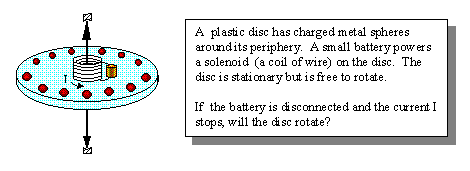"Do you remember the paradox we described in Section 17-4 about a solenoid and some charges mounted on a disc? It seemed that when the current turned off, the whole disc should start to turn. The puzzle was: Where did the angular momentum come from? The answer is that if you have a magnetic field and some charges, there will be some angular momentum in the field. It must have been put there when the field was built up. When the field is turned off, the angular momentum is given back. So the disc in the paradox would start rotating. This mystic circulating flow of energy, which at first seemed so ridiculous, is absolutely  necessary. There is really a momentum flow. It is needed to maintain the conservation of angular momentum in the whole world." (p. 27-11)

Of course, this is not a good way to make an electric motor. Motors must operate continuously. The illustrations simply show that motion of mass through space, and motion of space through mass have analogous behavior.

Want to get your students interested in STEM programs?  Have them look at these two photos:           and   #McCandlishDrawing   .  Ask them if a combination of electrical and magnetic  fields could be used to oppose gravity.  And what would be the response of Feynman's disk if  it were externally forced to rotate in the opposite direction (or held stationary) when the field was turned off? Would there be a momentary weight loss? (I don't know the answer to this question)

The physics of electromagnetism has a lot of concepts that seem strange and awkward on first encounter. The physics of space/time ratios and the concepts of multidimensional motion will, hopefully, allow you to become more comfortable with these concepts.

"Observation of Static Electromagnetic Angular Momentum in vacuo," Graham and Lahoz, Nature V. 285, May 15, 1980, p.129;  "Although this result is to be expected by classical electromagnetism, it leads inexorably to the acceptance of the physical reality of the Poynting vector, even though E and H arise from independent sources. . . . permanent magnets and electrets can be used to build a flywheel of electromagnetic energy steadily flowing in circles in the vacuum gap of a capacitor as if Maxwell’s medium were endowed with a property corresponding to superfluidity." http://www.tts.lt/~nara/introduc/introduc.htm

"Engineering the Zero-Point Field and Polarizable Vacuum for Interstellar Flight ", H.E. Puthoff*, S.R. Little, and M. Ibison: "This raises the issue as to whether static (i.e., non-propagating) ExH fields also constitute momentum (as the mathematics would imply), and in particular whether changes in static fields could result in the transfer of momentum to an attached structure. As it turns out, the answer can be yes as illustrated in the example of the Feynman disk paradox. . . . Even though nothing is apparently in motion, if we take the ExH momentum concept seriously, it would appear that there is angular momentum "circulating" about the disk in the static fields. . . ." http://www.stealthskater.com/Documents/Puthoff_1.pdf

Feynman Cylinder Paradox, John Belcher, Kirk T. McDonald (1983, 2002) http://www.physics.princeton.edu/~mcdonald/examples/feynman_cylinder.pdf

"Gyroscope's unexplained acceleration may be due to modified inertia", Lisa Zyga (July 26, 2011)
by http://phys.org/news/2011-07-gyroscope-unexplained-due-inertia.html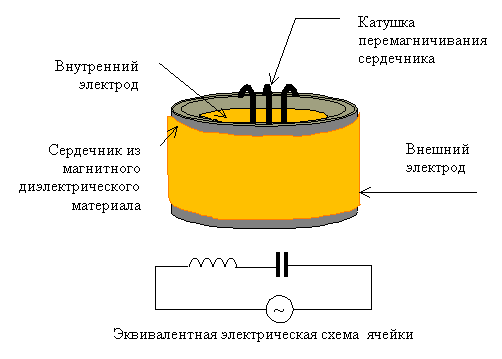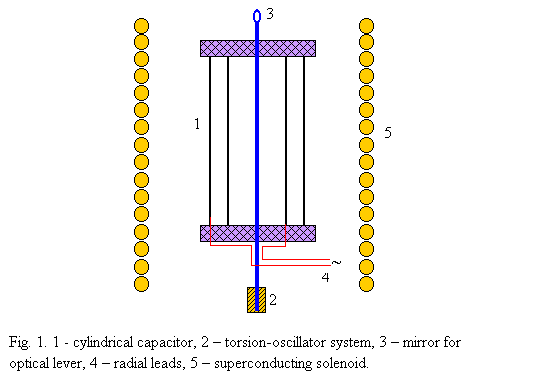http://www.tts.lt/~nara/amper/neutron.html                     http://www.tts.lt/~nara/introduc/introduc.htm (Grahm-Lahoz)

"Asymmetry and Energy in Magnetic Systems",
http://www.steorn.com/images/asymmetry-and-energy-in-magnetic-systems.pdf

"Magnetic Torque Measurement System"
http://www.steorn.com/images/magnetic-torque-measurement-system.pdf

"The problem of creating something which is new, but which is consistent
with everything which has been seen before, is one of extreme difficulty. "
(The Feynman Lectures on Physics, Vol. II, p. 20-10 to 20-11)

Space/time dimensions for some electromagnetic quantities

We saw previously  that energy is t/s, that mass and inductance are both  t3/s3, and that electrical current is s/t. It is possible to use these dimensions to derive other dimensions for electrical quantities. Power (energy per time), for instance,  is 1/s.

The less obvious dimensions can be derived from common electrical formulas. The voltage across and inductor, discussed above, is V = L(dI/dt) and we can use it to find the dimensions of voltage ("electric potential").  Hence,  V [=] t3/s3 (s/t)(1/t) or t/s2. We can also readily see that the formula is analogous to F = ma, where a = dv/dt. Voltage even has the same space/time dimensions as mechanical force.

Electric field intensity (E) is measured in volts per meter and therefore is t/s3. This can also be derived from formulas found in college level textbooks on electromagnetic science: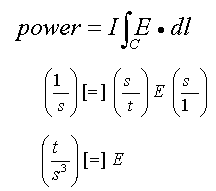In a similar way we can find the space/time dimensions for a magnetic field: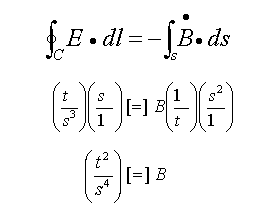Does E = cB agree with these dimensions?   We can readily see that it does.

Does it work for the Hertzian wave equation?: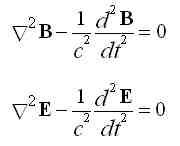Dimensions of  terms that are added or subtracted must be the same. Hence, for the first equation  (B):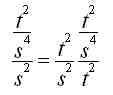which comes out to t2/s6 for both terms;    and for the second one (E):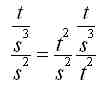which reduces to t/s5 for both terms.

_____

The magnetic vector potential (denoted as A) is also very important in electrodynamics. It has the dimensions of momentum per charge. That comes out as (t2/s2) (1/s) or t2/s3. The B field is supposed to be the curl (mathematically a spatial derivative) of the A field. That comes out as (t2/s3)(1/s) =  t2/s4 and is as shown above.

Incidentally, the magnetic vector potential is another one of those historical oddities:

"This subject has an interesting history. The theory we have described was known from the beginning of quantum mechanics in 1926. The fact that the vector potential appears in the wave equation of quantum mechanics . . . was obvious from the day it was written. That it cannot be replaced by the magnetic field in any easy way was observed by one man after the other who tried to do so . . . . It seems strange in retrospect that no one thought of discussing this experiment until 1956, when Bohm and Aharanov first suggested it and made the whole question crystal clear. The implication was there all the time, but no one paid attention to it. Thus many people were rather shocked when the matter was brought up. . . . It is interesting that something like this can be around for thirty years but, because of certain prejudices of what is and is not significant, continues to be ignored." (The Feynman Lectures on Physics, Vol. 2, page 15-12)

Because the vector potential A is regarded as more fundamental in quantum electrrodynamics "E and B are slowly disappearing from the modern expression of physical laws; they are being replaced by A and f ." (ibid., page 15-14)

"Thoughts on the Magnetic Vector Potential", John R. Taylor, 1996. http://www.scribd.com/doc/24546297/Thoughts-on-the-Magnetic-Vector-Potential-by-John-R-Taylor
"What the electromagnetic vector potential describes", E. J. Konopinski, 1977, http://physics.princeton.edu/~mcdonald/examples/EM/konopinski_ajp_46_499_78.pdf
"Apparatus for Generating Motional Electric Field", William J. Hooper, 1972.  http://www.rexresearch.com/hooper/3610971.htm
"New Biophysical Field Attacks Cancer Cells", Ivan Rampl, 2008. http://www.rampl.eu/

Simple demonstration of vector potential reality:

"If a wire is threaded through the hole of the toroid and returned through the hole, case 1, no galvanometer deflection is seen as B is switched on and off. But if the wire is threaded through the hole and returned around the coil, case 2, a galvanometer deflection will be seen from = -d/dt.  "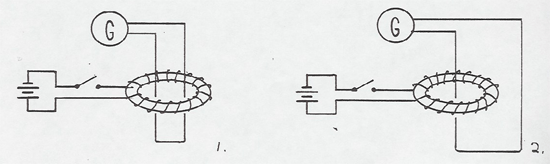http://pages.videotron.com/ceber/on_the_einstein-de_haas_and_barnett_effects.pdf ; http://encyclopedia2.thefreedictionary.com/Einstein-de+Haas+Effect
"The Connection Between Inertial Forces and the Vector Potential", Alexandre A. Martins,  Mario J. Pinheiro http://arxiv.org/ftp/physics/papers/0611/0611167.pdf ;

http://www.freepatentsonline.com/5845220.pdf   "Communication method and apparatus with signals comprising scalar and vector potentials without electromagnetic fields", Harold E. Puthoff   (12/01/1998)

One advantage of the present invention, alluded to before, is that the vector and scalar potentials penetrate electromagnetic shields. This is because electromagnetic shields work on the principle that incident (E, B) fields induce counter-effects that cancel said incident fields in the shielding materials. Such shielding effects do not occur for signals based solely on vector and scalar potential concepts, frequently referred to hereafter as (A, φ) signals. Devices based on the (A, φ) concepts are frequently referred to herein as pure-potential devices (PPD's).

A further advantage of the present invention is that prior art electromagnetic receivers do not detect pure-potential signals. The prior art electromagnetic receivers are based on detecting electric and/or magnetic fields, neither of which is present in devices, systems and methods in accordance with the present invention. Hence, the present invention provides an overlapping, parallel use spectrum that exists side by side with the ordinary electromagnetic spectrum. The pure-potential transmission does not interfere with the prior art electromagnetic transmission and vice versa.

It is, accordingly, an object of the present invention to provide a new communication method and apparatus.
__________

But there are some troubling inconsistencies in electromagnetic space/time dimensions. The Poynting vector is usually interpreted as power per area which comes out to 1/s3. The literature also expresses it as

S = gc2

where g is the momentum density (momentum per volume) and which has the dimensions of t2/s5. According to this formula then, S works out to be 1/s3, exactly as expected. But we are not so fortunate when expressing S in terms of E and B as in this formula:

S = e0c2E X B

The permittivity, e0, is expressed in coulombs per volt per meter. Charge is the one-dimensional analog of mass, and so permittivity is probably (t/s)(1/(t/s2))(1/s), which appears to be dimensionless. The dimensions for the remaining items have been given previously, and with those, S works out to be (s2/t2)(t/s3)(t2/s4) or t/s5. That is obviously NOT the same as 1/s3 which is what we were expecting. Did we mess up something when we used the vector cross product?  Does S have some alternative interpretation which would account for the discrepancy? Has a term inadvertently been left out of the formula?  Is a profound insight lurking here somewhere?

Perhaps the guess on the dimensions for permittivity were wrong. Simple inspection shows that they would have to be (s2/t) for the Poynting formula to work out correctly. Permittivity is used in formulas for capacitance, so maybe we can look at some capacitance formulas, explore the dimensions, and see if our new guess for the e0 dimensions is reasonable. Here is a good formula (Electrical Engineering Circuits, H.H. Skilling, 2nd ed., p. 18) to start with. It has obvious space/time dimensions and little else: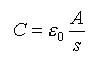C is the capacitance, e0 is the permittivity of free space (vacuum), A is the area of the plates, and s is the separation between plates. Plugging everything in, we find that the dimensions for capacitance (C)  are (s2/t)(s2)(1/s) which is just (s3/t) .

We now must find out if these dimensions for capacitance make sense. If they do, then the new choice for the permittivity dimensions is probably correct as well. Let's try the formula for current through a capacitor (note that v is voltage here, not velocity):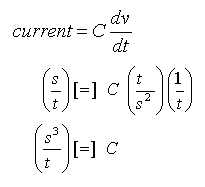This is perfect. We were hoping that the space time dimensions of capacitance would work out to be (s3/t) and that is exactly what we got. We can now confidently check other capacitance formulas and see if they to give the same dimensions for capacitance. Here is one that relates the charge on the capacitor plates to capacitance and voltage: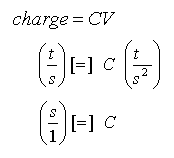And we find . . . that it doesn't work.  Instead of getting (s3/t), we get just (s).

Let's go back to the original capacitor formula:   C = e0 (A/s).   If  permittivity were dimensionless as originally supposed, the formula above,   charge = CV, would be dimensionally correct. In other words, capacitance is just a space unit.(s). If we take that value and plug it into the formula for current, we find that current is (s/t) instead of (t/s).  This seems to be trying to tell us something.

Rather than have two kinds of capacitance, it is more reasonable to believe that there are two kinds of electric current.   This has already been discussed elsewhere. One type of electricity, the so-called "static electricity",  is based on coulombic charge which has the dimensions of (t/s). Ordinary electric current, however, is just space per time (s/t), like a velocity, as discussed above and in the first section.  Maybe "storing current" in a capacitor is equivalent to stuffing it with space units. Or maybe is it equivalent to stuffing it with coulombic charge units. Which is it? The formula for force exerted on a charge by an electric field between the plates of a capacitor is F = QE . Force is (t/s2) and electric field is (t/s3).  It is obvious that the kind of Q (charge) we are looking at here must have the dimensions of (s) and not (t/s). In contrast to the inductor, what the capacitor stores is a "stopped" version of (s/t) or, in other words, just (s). And unlike the inductor, the electricity does not "squirt back out" when the battery is disconnected; a capacitor acts more like a storage tank than a pressurized accumulator (especially when the dielectric is a vacuum).

The conceptual problems here can be illustrated by thinking about a capacitor storing electrons as though it were a jar that stores marbles.  Note that the marbles are stored throughout the jar's volume, not just on the jar's surface, and that the jar has limited capacity.   If we want to store more marbles, we need to have a jar with more space. However, the marbles (electrons) represent space units  (rotational space). Putting space units into the jar, and then asserting that the jar is running out of space does not make any sense. We need a jar that has temporal volume instead of spatial volume.  That way, adding more electrons creates a space/time (or time/space) ratio. This ratio also implies a limited capacity.  But how do we put more temporal volume into a capacitor?  As explained previously, matter itself is a temporal structure (t3/s3). Putting a slab of matter between the two plates, creates more temporal volume, more "capacity". Some materials work better than others for this purpose, and this in turn leads to concepts like "capacitivity"something that is today called "permittivity".

Permittivity is thus an actual physical characteristic, and therefore should NOT be a dimensionless quantity. The second guess on the dimensions for permittivity (s2/t) is probably correct.

Anyway, the point here is that space/time dimensions for electromagnetic and mechanical quantities can be worked out, despite some obvious difficulties and long standing misconceptions. Ultimately, everything in physics can be expressed in terms of space/time ratios of some sort. This can lead to new insights about the true nature of these entities and their relationships with other entities.

For example: the electric field has the dimensions of  t/s3 (as shown above; ( t/s3 can also be energy density)). The second time derivative of that would be (t/s3) /t2  which comes out to  t3/s3 [?? (t/s3) /(1/t2) ?? math error ?*] , which has the same dimensions as mass. That implies that  a pulsed electric field could have a mass effect (a.k.a. "motional effect"). Could this be the real physics behind the Podkletnov Impulse Gravity Generator? See 3-30-11 Update and 6-14-03 Update  This invites even further speculation. Could a  magnetic "charge" ("flux", t2/s2) be somehow "physically" combined with an electric charge (t1/s1) to get a "mass field" (t3/s3 ) yielding a (temporary) motional effect?   Does the Biefeld-Brown effect through the Poynting vector and the magnetic and electric components of Feynman's carrousel (above) represent physical implementations of these relationships? [*the math here is wrong and this explanation cannot be valid.  The second time derivative,  d2 (t/s3)/dt2,  is akin to an acceleration of the electric field intensity. The first time derivative, d (t/s3)/dt,  is equivalent to 1/s3   which could be power/area . Neither of these alternatives seems to suggest a gravitational effect. However, power/area is the same dimension as the Poynting vector, which apparently has something to do with "ether power flow" (for lack of better words) and hence, gravitation. A spatially accelerated B field also has these dimensions:  B=t2/s4 as above, and a = s/t2 and so (B)(a) = (t2/s4)  (s/t2) which comes out to 1/s3 or power/area; however, this might require a homopolar configuration. What really seems to matter in all this is, not just power flow, but momentum  density. Says Feynman:

"There is an important theorem in mechanics which is this:  whenever there is a flow of energy in any circumstance at all (field energy or any other kind of energy), the energy flowing through a unit area per unit time, when multiplied by 1/c2, is equal to the momentum per unit volume in the space. In the special case of electrodynamics, this theorem gives the result that g is  1/c2 times the Poynting vector:

g = 1/c2 S

So the Poynting vector gives not only energy flow but, if you divide by  c2, also the momentum density."

In a charging cylindrical capacitor, or even a current in a resistance wire, the Poynting vector is directed radially inward, and therefore the momentum is likewise. The radial components balance out, and so in the case of the resistance wire, heat, not motion is the result. If the capacitor is asymmetrical the momentum components will not balance as in the radial case, and there will be 'leftover" momentum pointing in some direction.

There are two things to consider here:  First, the c2 factor suggests enormous electric and magnetic fields will be required for "motional effects". But momentum is also related to radiation reaction,  and that in turn, according to Feynman, is related to the third time derivative of position (something engineers and physicists call "jerk") of the electric charge used to produce the radiation. (Feynman, Vol 2, p. 28-7) The "back reaction" force on a radio antenna emitting 1 kW of radiation is equivalent to the weight of a few fleas. But these systems use sinusoidal acceleration fields and the "jerk" is relatively  mild, and the charge motion is cyclic.  In contrast, the fields in experiments giving levitation effects are described as very intense, unidirectional, and pulsed in such a way that the "jerk" on the charge has an extremely high value. (See Piggott example and Podkletnov Impulse Gravity Generator ; See also Poynting Vector Reversal )

Second, reconsider the example above with the static electric field and permanent magnet. Remember that the sources of the E and B fields can be independent. Feynman points out that the energy (and momentum) is just going around in circles. But a  circulating momentum is angular momentum.   So this is kind of like a flywheel, but it is constructed from the "ether" so to speak. To extract energy from it, something has to change, and this leads us back to Feynman's disk paradox where the magnetic field is turned off, and the disk experiences a rotational impulse.

Electromagnetic theory is still incomplete. Maxwell's equations do not include the Lorentz force, nor anything about the relation of gravity to electromagnetism. Additionally, there are still some very messy, difficult issues. Says Feynman:

"In bringing together relativity and Maxwell's equations, we have finished our main work on the theory of electromagnetism. . . . this tremendous edifice, which is such a beautiful success in explaining so many phenomena, ultimately falls on its face. . . . Now we want to discuss a serious trouble—the failure of the classical electromagnetic theory. . . . the classical theory of electromagnetism is an unsatisfactory theory all by itself. . . . when electromagnetism is joined to quantum mechanics, the difficulties remain. "  (The Feynman Lectures on Physics, Feynman, et al. (1964) Vol 2, p. 28-1)
_____

There are indications that the early post WWII UFOs used rotating magnetic fields in their propulsion systems.  One way to get spatially large magnetic effects would be to use intense, time-changing electric fields. One equation is E = cB.  It implies, due to the c factor, that the electric field intensity would have to be enormous to generate even a miniscule magnetic effect.  A time changing electric field induces a magnetic field, and so very fast time changes are also required.  This suggests a machine that uses fields of hundreds of millions of volts and switching times on the order of nanoseconds.  A van de Graaff generator with spark gap switching is probably the first thing that would come to mind; however, far more sophisticated machines of various designs can be built today.  And, in line with the above thinking, a spatially accelerated magnetic field could be produced by rotating the electric field by means of multiple electrodes and multiple switches. This suggests a design somewhat like that seen on a "drone" in California: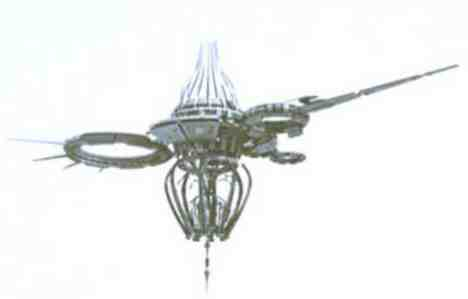http://www.youtube.com/watch?feature=endscreen&v=BXpVJnpu8Ac&NR=1   ;  For a discussion, see ADVPROP.html#StrangeFlyingMachines   .

Others have noted the relevance of rotating magnetic fields. Says physicist Eugene Podkletnov:   ( http://www.youtube.com/watch?feature=player_embedded&v=AgyAFElQZcU )  21:00 --> But to be absolutely honest now, after twelve or fifteen, already, years of research in this field, we came to the conclusion that it is not necessary to use superconducting materials in order to modify the gravity field. We can use rotating magnetic fields, and we can turn to normal conductors, which is much easier, much easier, and uh, ah, this method has a lot of advantages.”   http://portal.groupkos.com/index.php?title=Eugene_Podkletnov_portal

See also my views on UFOs in Make Sure of All Things

Books:

The Feynman Lectures on Physics, Vol. 2, Feynman, Leighton and Sands, 1964 http://www.scribd.com/doc/20698010/Vol-2-Ch-27-Field-Energy-and-Momentum

Basic Properties of Matter, Dewey B. Larson, 1988
New Light on Space and Time, Dewey B. Larson, 1965
Nothing But Motion, Dewey B. Larson, 1979

The Reciprocal Systemhttp://www.rsystem.org
The Collected works of Dewey B. Larson,  http://www.rsystem.org/dbl/index.htm

An update on the "electron as space" concept (6-14-2004)

The various discussions in the first article above have been based on the premise that an electron acts like a unit of actual space.  The presentation so far has included the following concepts:

1. Space moving through a wire partially cancels one dimension of gravitational motion and leaves a magnetic field as a residual effect.
2. Space moving through a wire has energy effects that are exactly analogous to the wire moving through space.
3. Space rotating in a coil of wire has angular momentum effects analogous to a coil of wire rotating
in space

We can now mention evidence for another concept:

4. Enormous electric current in a wire adds physical space between the atoms of the wire and may even cause the wire to tear apart. If the ends of the wire are securely clamped, the wire may buckle back upon itself.

Adding more space to a wire reduces its material density. In other words we would expect the wire to expand. If this could be done with extreme suddenness, the ordinary forces that keep the atoms of the wire together will be overcome, and the wire will be blown apart. However, the effect would be mechanical, rather than thermal; the wire does not melt or vaporize.

Evidence for just such an effect comes from experiments of Dr. Peter Graneau,  Dr. Jan Nasilowski and others.  They found that in electrodynamic wire explosions, the breaks were due to tensile stress (which act longitudinally) rather than pinch-off forces (which act radially). The wire seems to  pull itself apart into discrete fragments in these experiments.

A similar experiment was done with segments of a copper rod stacked vertically in a glass tube. When an enormous current pulse was sent through this stack, the copper segments moved apart. Photos showed multiple electric arcs between the segments.

Explanations for railgun recoil effects have led to similar conclusions. In these experiments the rails are securely anchored and have no freedom to expand. Sometimes the rails crumple back upon themselves as though there were tremendous unrelieved expansion forces. It is an effect similar to an asphalt road "washerboarding" on a hot summer day.

In conclusion:

"We have thus seen how solid conductors can bend and buckle, and even shatter, if subjected to high current pulses. The discussion indicates that some kind of longitudinal stress is present." , http://www.df.lth.se/~snorkelf/Longitudinal/node3.html , http://www.df.lth.se/~snorkelf/Longitudinal/node5.html http://www.df.lth.se/~snorkelf/LongitudinalMSc.pdf

You can see an example of this at http://www.magnet.fsu.edu/education/tutorials/slideshows/shrinkingquarter/index.html . A pulsed magnetic field is used to compress a U.S. coin to about 75% of its original diameter. Note the shards of copper wire after the experiment. The wire was blown apart, not melted, by 100,000  amps!__________

(I am waiting for the day when someone uses powerful electric and magnetic fields to cause a coin to "de-localize".  The coin will disappear entirely from the experimental setup.)

“A Bibliography of the Electrically Exploded Conductor Phenomenon,” by William G. Chace and Eleanor M. Watson, published by the Armed Services Technical Information Agency.http://www.dtic.mil/cgi-bin/GetTRDoc?AD=AD0299253&ei=_-awUrSVOJDyoASBhoD4Aw&

"Weber electrodynamics, part I. general theory, steady current effects", "Foundations of Physics Letters" 3(5):443-469 � October 1990 DOI: 10.1007/BF00665929  1st J. P. Wesley

"On the occurrence of ‘bead lightning’ phenomena in long laboratory sparks"
• S.P.A. Vayanganie, ,
• V. Cooray,
• Mahbubur Rahman,
• Pasan Hettiarachchi,
• Oscar Diaz,
• M. Fernando (Feb 2016)    http://www.sciencedirect.com/science/article/pii/S0375960115011184

•

If more space can be added to a material aggregate and result in a  decrease in density, then it is natural to also ask:   "Can more time be added to a material aggregate and result in an increase in density?"  If this can be done, then the possibility of producing materials with ultrahigh densities and attendant useful properties might become a reality.  Ultrahigh density diamond, for example, should be able to easily cut through normal diamond.

The possibility of making "high energy density materials" is of great interest to the military. Chemical explosives have specific energies of 5 MJ/kg. Thin film batteries and superconducting rings have about 1 to 50 MJ/kg. In chemical explosives the energy comes from chemical bonding, which is about 10eV per molecule. Superdense materials would have bond energies MUCH higher than this.

Conventional high explosives, for example,  have specific energies on the order of 5kJ/g and maximum mass densities of 2 g/cm3. This corresponds to an energy density of 10 kJ/cm3.   So-called "metallic hydrogen" is believed to have an energy density of about 270 kJ/cm3 which would be about 35 times more energetic than TNT. It could be used as a powerful "conventional" (non-nuclear) military explosive. A bomb containing one ton of metallic hydrogen would then be equivalent in destructive potential to 35 tons of ordinary TNT.  It could also be used to make nuclear weapons much more compact, possibly even obviating the use of a fission trigger (fission makes the bomb radioactively dirty, and the materials required for the fission trigger are very expensive and hard to obtain; a design with no fission trigger would make cost proportional to explosive power, but could also prove to be a proliferation nightmare).  As a rocket fuel, metallic hydrogen would have high specific impulse, say, 1400 sec compared  to 400 sec for JP4 + liquid oxygen. As an aircraft fuel it would have 300 times more energy than the best available, which implies a flight range 300 times normal.Astronomers are well aware of the existence of extremely high density matter, such as that in white dwarf stars. They also know about high density structures, such as compact, high velocity jets, and even compact galaxies. There is no generally accepted comprehensive theory explaining the "compactness" features of the universe.  With tongue in cheek I can say that it might just be a matter of time.

Fourth Generation Nuclear Weapons, Andre Gsponer, Jean-Pierre Hurni, 1999,  6th ed., p. 101 to 121;

Google "patent 905847 German Karl Nowak" to find information on experiments done in Nazi Germany on extreme densification of  materials (jet fuel, explosives, etc.). The method used high pressure compression and cryogenic cooling.

Science News, Vol. 157, Jan 22, 2000, p. 54 (octanitrocubane);

"Nitrogen Power: New crystal packs a lot of punch", Science News, July 17, 2004, Vol. 166, p.36-37, www.sciencenews.org/articles/20040717/fob4.asp )

"The Nuclear Threat That Doesn't Exist – or Does It?" by Sam Cohen and Joe Douglass, March 11, 2003
http://www.financialsense.com/editorials/douglass/2003/0311.htm   (comments on ballotechnics and red mercury)

Ray guns, Nuclear Isomers, Rydberg Atoms

http://www.aip.org/pnu/2004/690.html
"Why Do Transformers Hum?", Physics News Update, Number 690, June 30, 2004

html 4/04a

Press your Back button to go

OR